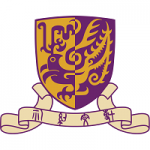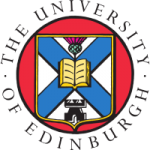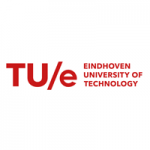# Mathematics

## 项目介绍

The Doctor of Philosophy (PhD) Program aims to prepare students to become research scholars in an academic or industrial environment and enable students to do independent and original research. It provides a broad background in mathematics and mathematical sciences. Students can choose to focus their research in one of the three areas: Pure Mathematics, Applied Mathematics, and Probability and Statistics. A candidate for a PhD degree is expected to demonstrate mastery of knowledge in the chosen discipline and to synthesize and create new knowledge, making an original and substantial contribution to the discipline.

The programs are offered by the Department of Mathematics with the following research foci and state-of-the-art facilities, strengthening students’ knowledge in mathematics and training them to carry out original research independently and innovatively.

Research Foci

Algebra and Number Theory

The theory of Lie groups, Lie algebras and their representations is an important branch in modern mathematics and it has interactions with many other branches in mathematics and physics. Our research includes representation theory of reductive groups, quantum groups, infinite dimensional Lie groups and Lie algebras and applications to mathematical physics. Number theory has a long and distinguished history, and the concepts and problems relating to the theory have been instrumental in the foundation of a large part of mathematics. Number theory has flourished in recent years, as made evident by the proof of Fermat’s Last Theorem. Our research specializes in automorphic forms and applications of number theory.

Geometry and Topology

Geometry and topology provide an essential language describing all kinds of structures in nature. The subject has been vastly enriched by close interaction with other mathematical fields and with fields of science such as physics, astronomy and mechanics. The result has led to great advances in the subject, as highlighted by the proof of the Poincare conjecture. Active research areas in the department include algebraic geometry, differential geometry, low-dimensional topology, equivariant topology, combinatorics and combinatorial topology, and geometrical structures in mathematical physics.

Analysis and Differential Equations

The analysis of real and complex functions plays a fundamental role in mathematics. This is a classical yet still vibrant subject that has a wide range of applications. Differential equations are used to describe many scientific, engineering and economic problems. The theoretical and numerical study of such equations is crucial in understanding and solving problems. Our research areas include complex analysis, exponential asymptotics, functional analysis, harmonic analysis, wavelets analysis, nonlinear equations and dynamical systems, integrable systems, fluid dynamics, and inverse problems.

Applied and Computational Mathematics

The research focuses on development of mathematical models and advance numerical algorithms, as well as applications of mathematics to interdisciplinary science and engineering areas. The research areas include: modeling and simulation in fluid dynamics and materials science, computational fluid dynamics, multiscale modeling, kinetic theory, atomistic simulations, image processing, computational harmonic analysis, inverse problems and wave propagation, graph theory, optimization, evolutionary genetics, environmental science, numerical weather prediction, ocean and coastal modeling, numerical PDEs and numerical linear algebra, parallel algorithms, and numerical software.

Financial Mathematics

Traditionally, financial mathematics includes three areas: asset allocation/management, derivative modeling, pricing and hedging, and division or firm-wise risk management. The mathematical tools include probability, stability, stochastic calculus and numerical analysis. The recent trend of financial mathematics research, however, has been data driven, parallel to the development in the financial market where both sell-side and buy-side firms are trying to adopt the methodologies of big data and machine learning, in order to gain advantages in proprietary trading, market making, hedging and investments. Some faculties of the department working in related fields have embraced the changes and adopted the new technologies in their teaching and research.

Probability and Statistics

Statistics, the science of collecting, analyzing, interpreting, and presenting data, is an essential tool in a wide variety of academic disciplines as well as for business, government, medicine and industry. Our research is conducted in four categories. Time Series and Dependent Data: inference from nonstationarity, nonlinearity, long-memory behavior, and continuous time models. Resampling Methodology: block bootstrap, bootstrap for censored data, and Edgeworth and saddle point approximations. Stochastic Processes and Stochastic Analysis: filtering, diffusion and Markov processes, and stochastic approximation and control. Survival Analysis: survival function and errors in variables for general linear models. Probability current research includes limit theory.

Data Science

Statistics and data science play a more and more important role in scientific research and applications, particularly in the era of big data. Some research interests include random matrix, survival analysis, financial statistics, econometrics, bioinformatics, statistical machine learning, statistical genetics and genomics, computational biology and bioinformatics, error in variables model, generalized linear models; biological and medical statistics, topological and geometric methods in data analysis.

We also develop algorithms that provably and significantly improve the state-of-the-art for mathematical data analysis, in terms of accuracy, running time, memory efficiency, scalability, or other computational measures. This includes adapting existing algorithms or approaches to exploit contemporary computing architectures, new statistical paradigms, or heterogeneous information sources. We investigate the solution of specific practical data science problems that use novel methodology and are of broad interest. These problems include imaging, high dimensional data analysis, machine learning, social networks, genetics and genomics.

Facilities

The Department enjoys a range of up-to-date facilities and equipment for teaching and research purposes. It has two computer laboratories and a Math Support Center equipped with 100 desktop computers for undergraduate and postgraduate students. The Department also provides an electronic homework system and a storage cloud system to enhance teaching and learning.

To assist computations that require a large amount of processing power, a High Performance Computing (HPC) laboratory was setup in 2000. The high-speed computers in this laboratory in which InfiniBand technology and GPU technology are applied are capable of delivering a processing power of 150 TFLOPS practically. By making use of these powerful computing facilities, our faculty and postgraduate students are able to solve computationally intensive problems in their innovative research projects so that they can stay at the forefront of their fields.

### 项目概览## 相关项目推荐

KD博士实时收录全球顶尖院校的博士项目，总有一个项目等着你！

•# Mathematical Modelling, Analysis and Computation

•# Geometry and Topology

•